### Home > CCA2 > Chapter Ch7 > Lesson 7.2.2 > Problem7-129

7-129.
1. Find an equation for each graph below. Homework Help ✎

2.  a.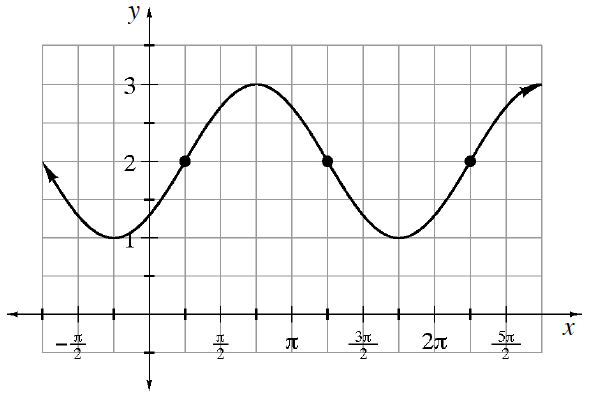b.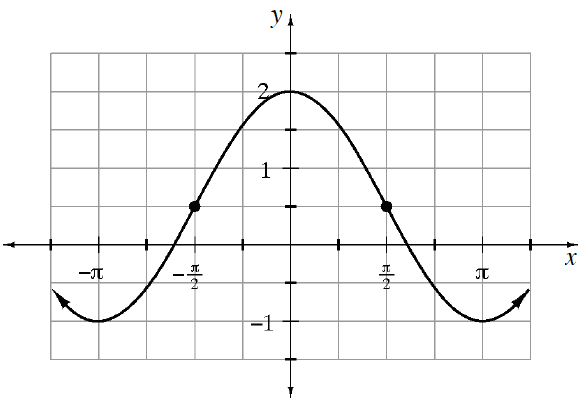c.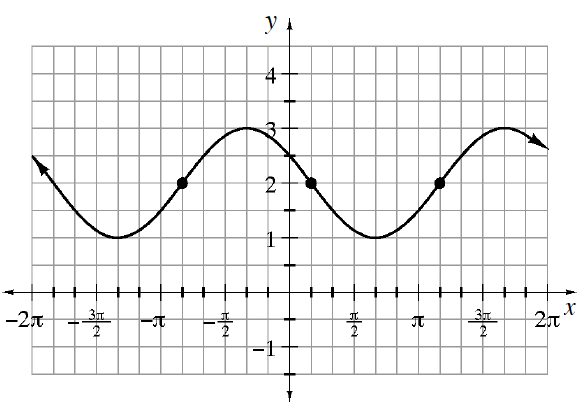d.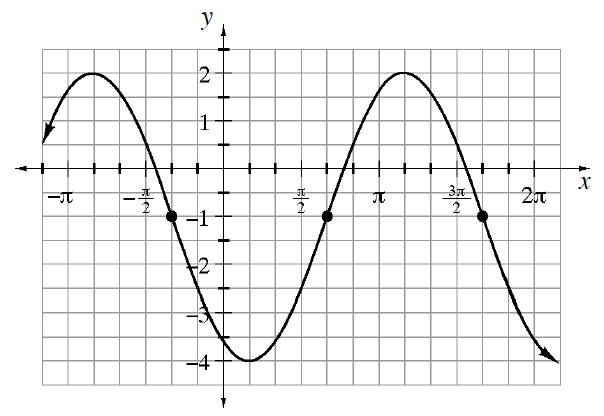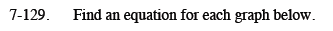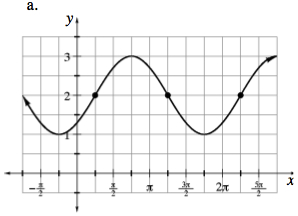Use the general equation y = a · sin(xh) + k.
Determine the value for each of the three parameters.

First identify a convenient locator point.
In this case, we will use:

$\left(\frac{\pi}{4}, 2\right)$

The x-value of the point represents the horizontal shift.
The y-value of the point represents the vertical shift.

$h=\frac{\pi}{4},k=2$

The amplitude (a) is the distance from the midline (k) to the highest point.
In this case k = 2 and the highest point is 3, so a = 1.
Since the graph increases from the locator point in the same way y = sin x does, a is positive.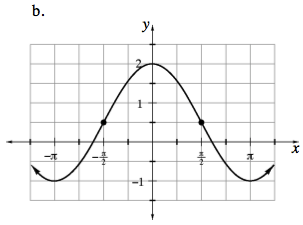See part (a).
Pay careful attention to the scale on the y-axis when determining a and k.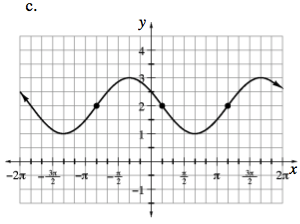$\text{If you choose } \left(\frac{\pi}{6},0\right) \text{ as your locator point,}$

notice the first cycle of the graph is an inverted sine curve, so the a value will be negative.

See part (a).

$y=-\sin\left(x-\frac{\pi}{6}\right)+2\text{ or }y=\sin\left(x+\frac{5\pi}{6}\right) +2$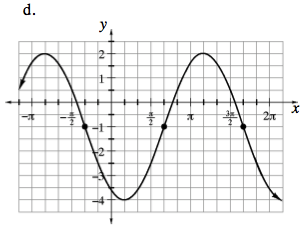See parts (a) and (c).